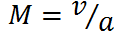# Mach Number Calculator

This CalcTown calculator calculates the Mach number of an object, i.e the number of times the object is moving with respect to sound.

*Please enter a=330 m/s for T=300 K and if nothing else is specified.

m/s
m/s

#### Resultwhere

v=Velocity of the Object

a=Velocity of sound